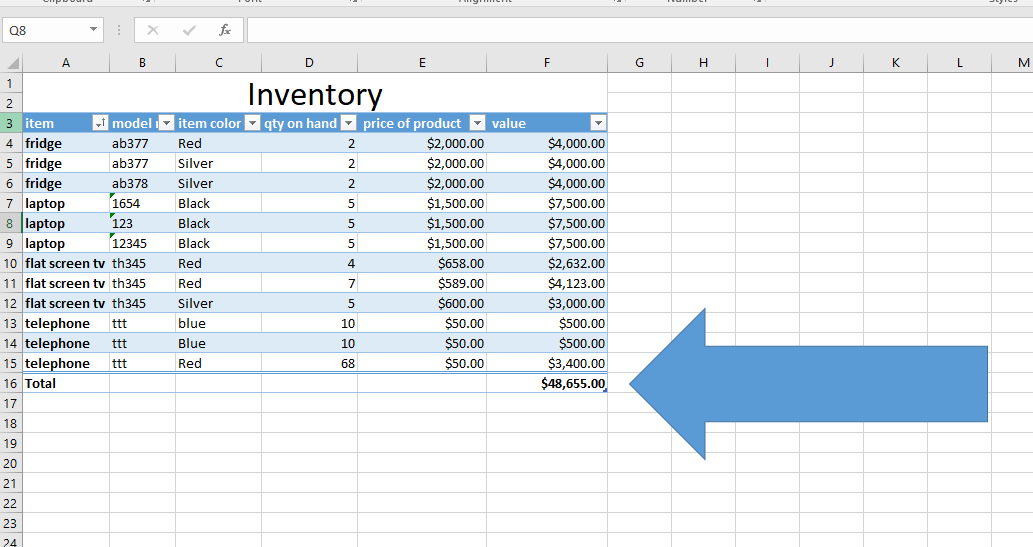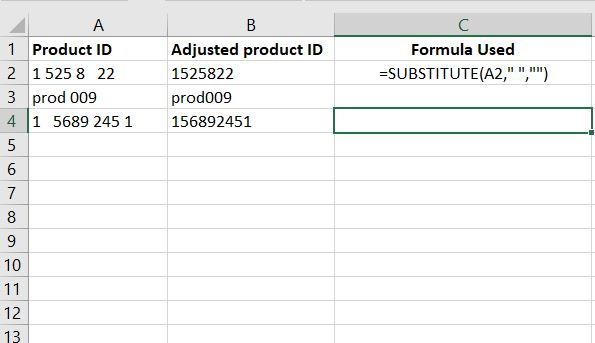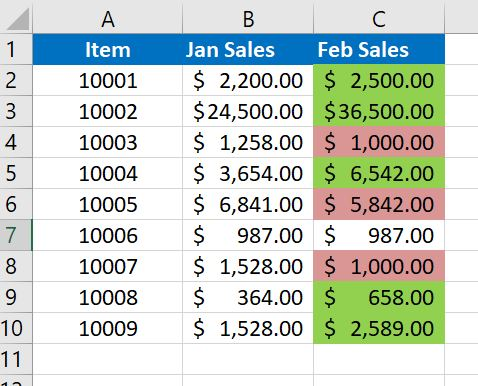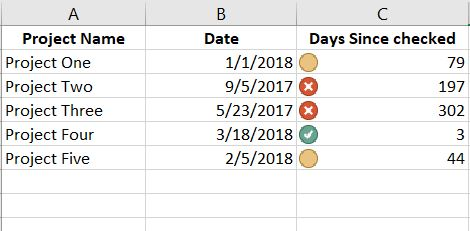## How to add a call out Arrow to an Excel SpreadsheetHow to add a call out Arrow to an Excel Spreadsheet Lets pretend that you have an Excel Spreadsheet with an important number that you do not want your viewers to miss. How do you add a call out arrow to your spreadsheet? Below is our sample spreadsheet with a total under the last row […]

## How to calculate age in ExcelHow do you calculate age using a formula or function in Excel To calculate age in Excel is relatively easy if you know the proper function to use. In this quick example I am going to use the today() function to perform some simple date math. In the below example we have a column of […]

## Missing Row and Column Headings in ExcelMissing Row and Column Headings This is a question I received for the first time during my latest Excel 2016 Class. My student mentioned that she had missing row and column headings on her spreadsheet. Although this is not a problem with Excel or error with the spreadsheet itself, new users may not be aware […]

## Excel Tips and Helpful Info for StudentsExcel Information and Tips Excel is an extremely powerful program. Most users do not take advantage of all of the capabilities Excel has to offer. Below, please find some links to some of the more helpful Excel Tips I have found. Please note I did not create the articles below. I am just linking to […]

## How to create an Excel Forecasting WorksheetIn this short example I am going to show you how to create an Excel Forecasting Worksheet to graph out with a certain percentage of accuracy a forecast of future number of calls. This example uses Excel 2016. This example is going to start with some historical data for the past 6 years worth of […]

## How to remove spaces in cell data in ExcelHow to remove spaces in cell data in Excel This came up in the last Excel class. A student asked how to remove spaces in Excel cell data. For example a product number that was entered incorrectly. In the below simple example we are going to use the SUBSTITUTE() fucntion in Excel to substitute a space […]

## Excel – Conditional Formatting ExampleExcel – Conditional Formatting Example I have been asked by a former student for some help with Excel’s Conditional Formatting. My student needed help setting up a column of data with a cell fill color, red or green, based on wether the previous column was lower or higher.  Here is the Excel – Conditional Formatting […]

## Excel Pivot Table ExampleExcel Pivot Table Example Many students and other Excel users often ask me what a pivot table is in Excel. Most users really have never needed them. It really is a rather useful tool if you know how to use it. I have put together this small Excel Pivot Table Example to help students understand […]

## Excel vLookup ExampleExcel vLookup Example While teaching Excel classes over the past few semesters I get many questions on how to perform certain fuctions in Excel. Below is another request for and Excel vLookup example. This is for a student that needed this for real world work. My student had two lists of customers. The first was […]

## Excel Date CalculationsHow to use simple Excel Date Calculations Several students have asked about Excel Date Calculations. Here is a good quick example to calculate the difference between todays date ( “today()”) and a date entered in a cell. For a real world example, I use this to track the time I follow up on small projects. For […]